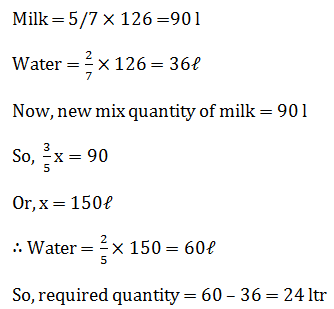# SSC Quantitative Aptitude Questions (Day-74)

Dear Aspirants, you can find the Quantitative Aptitude questions with detailed explanations for the SSC exams. Nowadays the competitive level of the exam has been increasing consistently. Due to the great demand for the government job, the level of the toughness reached greater. Candidates have to enhance the preparation process in order to drive in the right path. It doesn’t need to clear the prescribed cutoff. You must have to score good marks more than the cut off marks to get into the final provisional list. Here we have updating the Quantitative Aptitude questions with detailed explanations on a daily basis. You can practice with us and measure your level of preparation. According to that you can sculpt yourself in a proper way. SSC aspirants kindly make use of it and grab your success in your career.

Start Quiz

1) What will be the remainder when 141 × 142 × 143 is divided by 6?

(a) 0

(b) 2

(c) 4

(d) 5

2) If (3 + 2√5)2 = 29 + K√5, then what is the value of K?

(a) 12

(b) 6

(c) 29

(d) 39

3)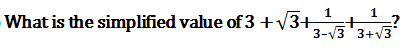(a) 2 + √3

(b) 2 – √3

(c) 4 – √3

(d) 4 + √3

4) If x = 5 + 2
6, then what is the value of x+1/√x?

(a) 2√3

(b) 3√2

(c) 2√6

(d) 6√2

5) Which of the following relation(s) is/are true?

I) 333 > 333

II) 333 > 333

III) 333 > 333

(a) Only I and II

(b) Only II and III

(c) Only II

(d) All I, II and III

6) 24 women can complete a work in 45 days. If 18 women are employed, then in how many days the work would be completed?

(a) 60

(b) 70

(c) 85

(d) 75

7) A and B undertook a work for Rs 2200. A alone can do the work in 10 days and B alone can do the work in 12 days. If they work together, then what will be the difference (in Rs.) in the amount they receive?

(a) 350

(b) 250

(c) 200

(d) 300

8) If 20% of marked price is equal to 30% of cost price. If no discount is given, then what will be the profit percentage?

(a) 50

(b) 40

(c) 48

(d) 45

9) Which of the following successive discount is the best for the customer?

I) 25%, 25%

II) 10%, 40%

III) 20%, 30%

(a) Only I

(b) Only II

(c) Only III

(d) All are same

10) In a mixture of 126 litre the ratio of water and milk is 2 : 5 respectively. How much water (in litre) must be added to make the ratio of water and milk as 2 : 3 respectively?

(a) 16

(b) 24

(c) 18

(d) 20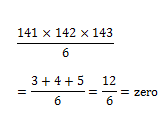= 9 + 20 + 12√5

= 29 +  k√5

So, k = 12

3 + √3 + 6/6 = 4 + √3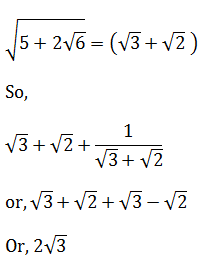333 = (33 )11 = (27)11

= 279.729

Clearly, 333 > 333

(as 729>333)

So, statement III is true

& 333 = 33.1089

Clearly, 333 > 333

So, statement II is correct.

& 278.19683 > 33.1089

∴ Statement I is correct

So, I, II & III all are correct

24 × 45 = 18 × x

12 × 5 = 60 = x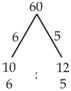∴ Difference = 1/11 × 2200

= 200

0.2MP = 0.3CP

MP = 1.5CP

∴ Profit % = 50%

I) 25 + 25 – 6.25 = 43.75%

II) 10 + 40 – 4 = 46%

III) 20 + 30 – 6 = 44%

Clearly, II is the best.